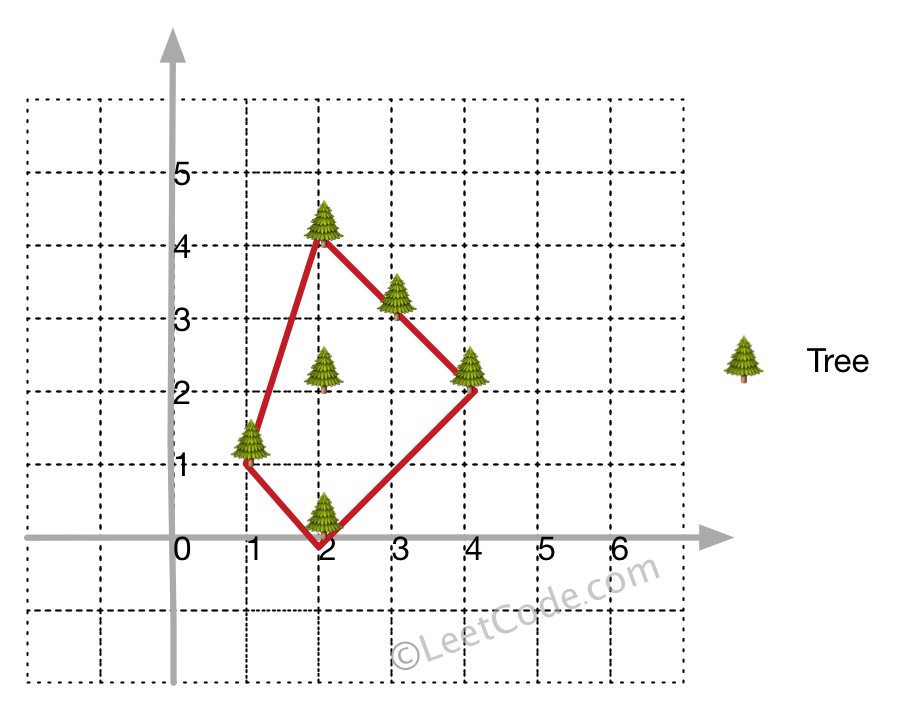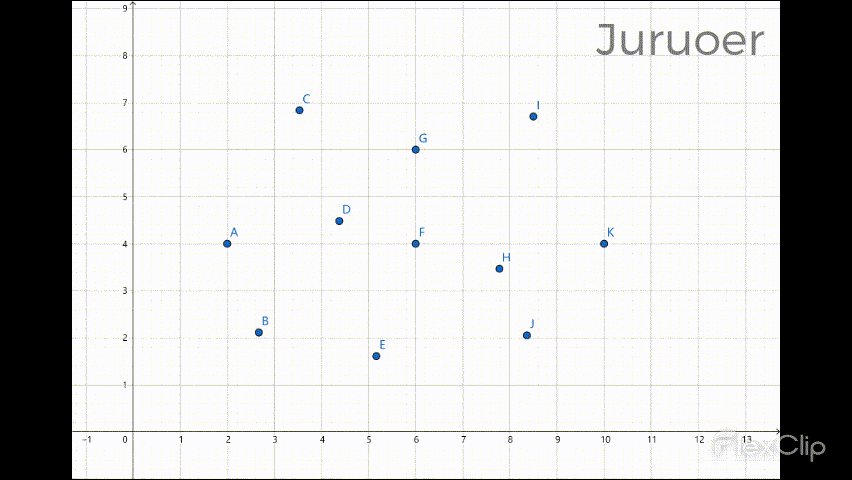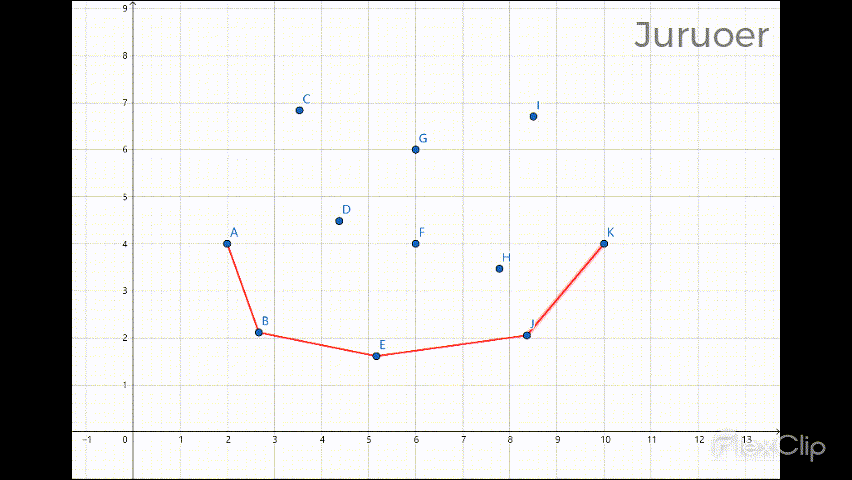## 二、题目描述• 所有的树应当被围在一起。你不能剪断绳子来包围树或者把树分成一组以上。
• 输入的整数在 0 到 100 之间。
• 花园至少有一棵树。
• 所有树的坐标都是不同的。
• 输入的点没有顺序。输出顺序也没有要求。

## 三、解决方案

### 2、前置知识

\begin{aligned} cross(p, q, r) &= \vec{pq} × \vec{qr} \\ &= \begin{vmatrix} (q_{x} - p_{x} ) & (q_{y} - p_{y}) \\ (r_{x} - q_{x}) & (r_{y} - q_{y}) \end{vmatrix} \\ &= (q_{x} - p_{x} ) \times (r_{y} - q_{y}) - (q_{y} - p_{y}) \times (r_{x} - q_{x}) \end{aligned}

### 3、Andrew算法

Andrew的办法是将所有的点按照x坐标从小到大排序，若x坐标相同则按y坐标从小到大排序，设排序后的点集为sortX，按照凸包的定义可以知道x坐标最小的点也就是sortX一定属于凸包。

1. 若栈中元素个数少于2，直接将r入栈，遍历下一个元素，否则进入2。
2. 取出栈中倒数第二个元素 p 和最后一个元素 q ，那么若cross(p, q, r)小于0，说明 r 位于$\vec{pq}$ 的右边，则 q 不满足凸包的定义（若q属于凸包，所有的点都应当在$\vec{pq}$ 的左边），将 q 出栈，进入1，否则将r入栈，遍历下一个元素。1. 若该点已经存在于下半区了，跳过该点，遍历下一个元素，否则进入2。
2. 若栈中元素个数少于m + 1，直接将r入栈，遍历下一个元素，否则进入3。
3. 取出栈中倒数第二个元素 p 和最后一个元素 q ，那么若cross(p, q, r)小于0，说明 r 位于$\vec{pq}$ 的右边，则 q 不满足凸包的定义，将 q 出栈，进入2，否则将r入栈，遍历下一个元素。